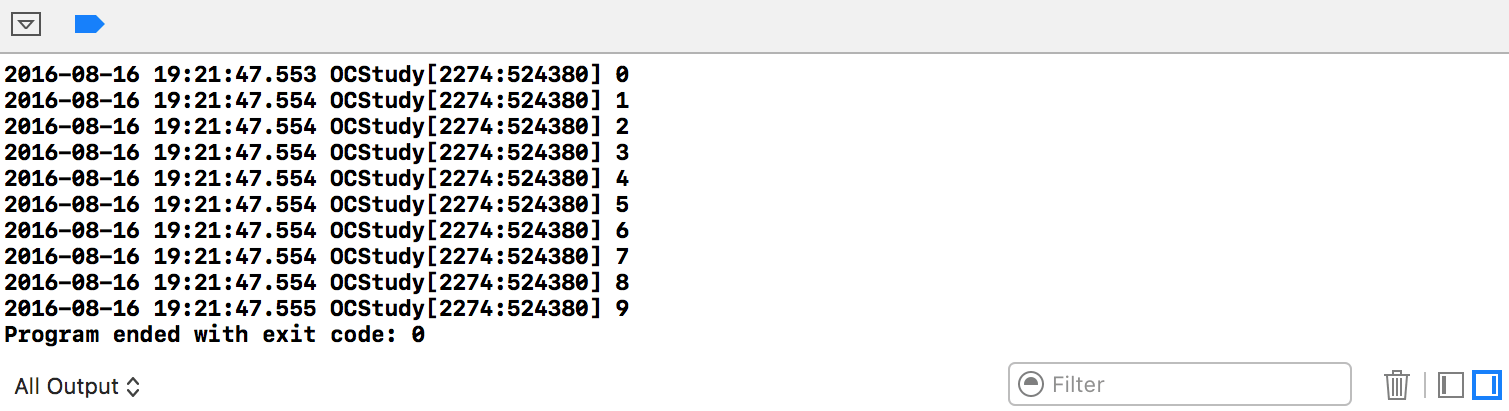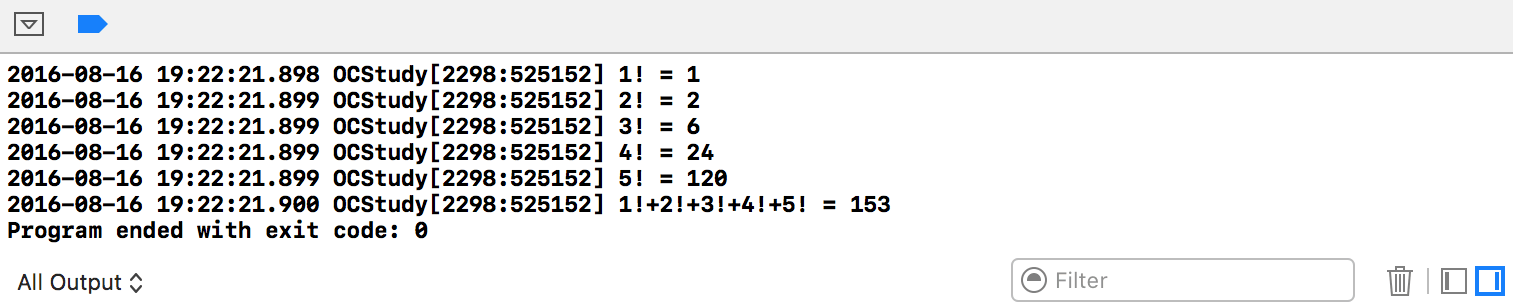# for循环简介

for循环是最常使用的循环方式。for循环是开界的，它的一般形式为：

``````for(<初始化> ; <条件表达式>; <增量定义> ) {
//循环执行的语句
...
}
``````

• 初始化总是一个赋值语句, 它用来给循环控制变量赋初值；
• 条件表达式是一个关系表达式, 它决定什么时候退出循环；
• 增量定义循环控制变量每循环一次后按什么方式变化。

``````for(i=1; i<=10; i++){
//循环执行的语句
...
}
``````

# for循环的实现形式

• 简单的for循环。下方的代码实现了打印0~9这10个数字。
``````int main(int argc, const char * argv[]) {
@autoreleasepool {
for (int i = 0; i < 10; i++) {
NSLog(@"%d", i);
}
}
return 0;
}
``````• 嵌套的for循环。示例代码中实现了求解从1到5五个数字先计算每个数字的阶乘，然后再累加求和。
``````int main(int argc, const char * argv[]) {
@autoreleasepool {
// 求1!+2!+3!+4!+5!的值
int sum = 0;
// 外层循环控制循环次数
for (int i = 1; i < 6; i++) {
int factorial = 1;
// 内层循环求i的阶乘
for (int j = 1; j <= i; j++) {
factorial *= j;
}
sum += factorial;
NSLog(@"%d! = %d", i, factorial);
}
NSLog(@"1!+2!+3!+4!+5! = %d", sum);
}
return 0;
}
``````# for循环的循环条件

``````for (int i = 0, j = 100; i < 10; i++, j--) {
...
}
``````

``````for (; j < 10; j++) {
...
}
``````

# 示例代码

https://github.com/99ios/2.3.1